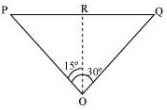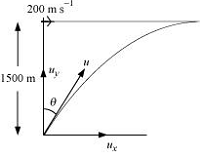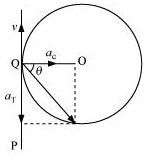# Topic: Motion in A Plane (Test 4)

Topic: Motion in A Plane
Q.1
A cricketer can throw a ball to a maximum horizontal distance of 100 m. How much high above the ground can the cricketer throw the same ball?
A. 76 m
B. 60 m
C. 65 m
D. 50 m
Explaination / Solution:

Maximum horizontal distance, R = 100 m
The cricketer will only be able to throw the ball to the maximum horizontal distance when the angle of projection is 45°, i.e., θ = 45°.
The horizontal range for a projection velocity v, is given by the relation:
R =( u2 Sin 2θ / g)
100 =
=> $\frac{{u}^{2}}{g}$= 100   ….(i)
The ball will achieve the maximum height when it is thrown vertically upward. For such motion, the final velocity v is zero at the maximum height H.
Acceleration, a = –g
Using the third equation of motion:
v2 – u2 = -2gH

Workspace
Report
Q.2
A stone tied to the end of a string 80 cm long is whirled in a horizontal circle with a constant speed. If the stone makes 14 revolutions in 25 s, what is the magnitude and direction of acceleration of the stone?
A. 10.2 m , along the radius at every point towards the center
B. 11.9 m , along the radius at every point away from the center
C. 9.9 m , along the radius at every point towards the center
D. 9.9 m , along the radius at every point away from the center
Explaination / Solution:

Length of the string, l = 80 cm = 0.8 m
Number of revolutions = 14
Time taken = 25 s
Frequency,
Angular frequency,

Centripetal acceleration, ac = ω2r

= 9.9 ms-2
The direction of centripetal acceleration is always directed along the string, towards the centre, at all points.

Workspace
Report
Q.3
An aircraft executes a horizontal loop of radius 1.00 km with a steady speed of 900 km/h. Compare its centripetal acceleration with the acceleration due to gravity.
A. 10 g
B. 6.4 g
C. 5 g
D. 23 g
Explaination / Solution:

Radius of the loop, r = 1 km = 1000 m

Speed of the aircraft, v = 900 km/h

Centripetal acceleration,

Acceleration due to gravity, g = 9.8 m/s​​​​​​​​​​​2

= 6.4 g

Workspace
Report
Q.4
Which of the following statements not true?
A. The acceleration vector of a particle in uniform circular motion averaged over one cycle is a null vector
B. The net acceleration of a particle in uniform circular motion is always along the radius of the circle towards the centre
C. The velocity vector of a particle at a point is always along the tangent to the path of the particle at that point
D. The net acceleration of a particle in circular motion is always along the radius of the circle towards the centre
Explaination / Solution:

The net acceleration of a particle in circular motion is along the radius of the circle towards the centre Only in case of uniform circular motion.

Workspace
Report
Q.5
The position of a particle is given by  Find the velocity and acceleration of the particle.
A. 3.0i^−4.0tj^ , -4.0j^
B. 4.0i^−4.0tj^ , -3.0j^
C. 2.0i^−4.0tj^, -2.0 j
D. 5.0i^−4.0tj^, -3.0 j
Explaination / Solution:

Position vector

We know velocity is given by

So

Acceleration is given by

So,

Workspace
Report
Q.6

A particle starts from the origin at t = 0 s with a velocity of 10.0  m/s and moves in the x-y plane with a constant acceleration of ( 8.0+ 2.0) m . At what time is the x- coordinate of the particle 16 m? What is the y-coordinate of the particle at that time?

A. 2.2 s, 22 m
B. 2 s, 24 m
C. 1 s, 14 m
D. 1.5 s, 20 m
Explaination / Solution:

u = uxi + uyj = 10j,

ux = 0 and uy = 10 m/s

a = axi + ayj = 8i + 2j,

ax = 8 and ay = 2 m/s2

x = uxt +axt2

=> 16 =

t = 2 sec

y = uyt +   = 10x2 + = 24 m

Workspace
Report
Q.7
An aircraft is flying at a height of 3400 m above the ground. If the angle subtended at a ground observation point by the aircraft positions 10.0 s apart is 30${}^{\circ }$, what is the speed of the aircraft?
A. 172 m/s
B. 192 m/s
C. 193 m/s
D. 182 m/s
Explaination / Solution:

The positions of the observer and the aircraft are shown in the given figure.Height of the aircraft from ground, OR = 3400 m
Angle subtended between the positions, ∠POQ = 30°
Time = 10 s
In ΔPRO:
tan 15° =
PR = OR tan 15°

ΔPRO is similar to ΔRQO.
∴PR = RQ
PQ = PR + RQ
= 2PR = 2 × 3400 tan 15°
= 6800 × 0.268 = 1822.4 m
∴ Speed of the aircraft =

= 182.24 m/s  m/s

Workspace
Report
Q.8

A fighter plane flying horizontally at an altitude of 1.5 km with speed 720 km/h passes directly overhead an anti-aircraft gun. At what angle from the vertical should the gun be fired for the shell with muzzle speed 600 m  to hit the plane? At what minimum altitude should the pilot fly the plane to avoid being hit ? (Take g = 10 m).

A. at an angle of 20.5 with the vertical, 12 km
B. at an angle of 23.5${}^{\circ }$ with the vertical, 17 km
C. at an angle of 21.5${}^{\circ }$ with the vertical, 15 km
D. at an angle of 19.5${}^{\circ }$ with the vertical, 16 km
Explaination / Solution:

Height of the fighter plane = 1.5 km = 1500 m
Speed of the fighter plane, = 720 km/h = 200 m/s
Let θ be the angle with the vertical so that the shell hits the plane. The situation is shown in the given figure.Muzzle velocity of the gun, u = 600 m/s
Time taken by the shell to hit the plane = t
Horizontal distance travelled by the shell = uxt
Distance travelled by the plane = vt
The shell hits the plane. Hence, these two distances must be equal.
uxt = vt
u Sin θ = v
Sin θ =
θ = Sin-1(0.33) = 19.50

In order to avoid being hit by the shell, the pilot must fly the plane at an altitude (H) higher than the maximum height achieved by the shell.
∴ H =

= 16006.482 m
≈ 16 km

Workspace
Report
Q.9
A cyclist is riding with a speed of 27 km/h. As he approaches a circular turn on the road of radius 80 m, he applies brakes and reduces his speed at the constant rate of 0.50 m/s every second. What is the magnitude and direction of the net acceleration of the cyclist on the circular turn?
A. 0.84 m , 58.5with the direction of velocity
B. 0.82 m , 59.5with the direction of velocity
C. 0.86 m , 54.5with the direction of velocity
D. 0.85 m , 56.5with the direction of velocity
Explaination / Solution:

Speed of the cyclist, v = 27 km/h = 7.5 m/s
Radius of the circular turn, r = 80 m
Centripetal acceleration is given as:
ac =  0.7 ms-2

The situation is shown in the given figure:Suppose the cyclist begins cycling from point P and moves toward point Q. At point Q, he applies the breaks and decelerates the speed of the bicycle by 0.5 m/s2.

This acceleration is along the tangent at Q and opposite to the direction of motion of the cyclist.
Since the angle between ac and aT is 900, the resultant acceleration a is given by:
a =
$\sqrt{0.74}$ = 0.86 ms-2

tan θ =
where θ is the angle of the resultant with the direction of velocity.
tan θ = = 1.4
θ = tan-1(1.4) = 54.56° with the direction of velocity

Workspace
Report
Q.10

A ball is thrown upwards with an initial velocity of 10 m . Determine the maximum height reached above the thrower’s hand. Determine the time it takes the ball to reach its maximum height.

A. 5.43 m, 0.92 s
B. 5.23 m, 1.12 s
C. 5.10 m, 1.02 s
D. 5.25 m, 0.42 s
Explaination / Solution:

Initial velocity u = 10m/s

As at the maximum height ball ll stop so final velocity v = 0 m/s

Only acceleration working on it is acceleration due to gravity g = -9.8m/s​​​​​​2

Let height = h

So we know

=>

=> $h=$ 5.10 m

Also let time taken to reach maximum height = t

Then

We know

v = u +at

=> 0 = 10 +(-9.8)t

=> t =

Workspace
Report